### Born-Oppenheimer approximation

In the conventional method for a molecular system, known as the Born-Oppenheimer approximation, nuclear motions are decoupled from electronic (or muonic) motions, i.e., the total wave functions are assumed to have the form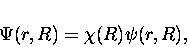(16)

where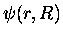, which is obtained from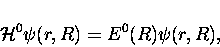(17)

are the eigenfunctions for the Hamiltonian for fixed nuclei,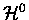, with E0 its eigenvalues. Here, the internuclear distance R is just a parameter. The nuclear wave function,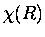, is solved using E0(R) as the effective potential for the nuclear motion;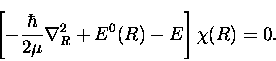(18)